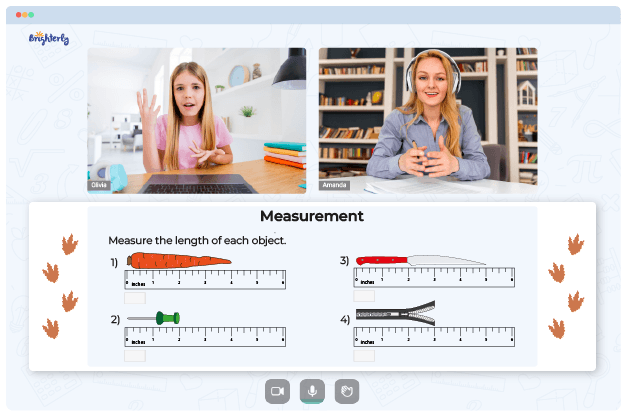# Measurement Conversion Worksheets

Kids should master measurement conversion as it is used in everyday activities. Students can use hands-on activities in metric measurement conversion worksheet that are relevant and engaging.

## How It Works

The converting measurements worksheet helps kids go through the process of converting a measurement from one unit of measure to another. To practice this skill, children can use a worksheet that includes a variety of objects with known measurements. To convert a height, they need to know the conversion factor and the number they will multiply the original size by to convert it to the new unit.

Math for Kids

Is Your Child Struggling With Math?
1:1 Online Math TutoringFor example, to convert inches to centimeters, they would multiply the number of inches by 2.54, which is the conversion factor for inches to centimeters. Understanding the concept of conversion can also help know how to convert a length from inches to centimeters or weight from pounds to kilograms.

## Metric System Measurement Conversion Worksheet Answers Benefits### Measurement Conversion Worksheets PDF

Converting Measurements Worksheet### Measurement Conversion Worksheets PDF

Metric Measurement Conversion Worksheet### Measurement Conversion Worksheets PDF### Measurement Conversion Worksheets PDF

Metric System Measurement Conversion Worksheet Answers

Measurement conversion worksheets contain activities that help kids build some essential conversion skills. Converting measurements requires children to understand and apply mathematical concepts, such as ratios, proportions, and rates. In addition to these practical benefits, measuring units worksheet convert answers provides kids with mathematical skills essential for understanding and using different measurement systems.

### More Measurement Worksheets

Struggling with Measurement?• Start lessons with an online tutor.

Is your child finding it challenging to grasp measurement concepts? An online tutor could be the answer.